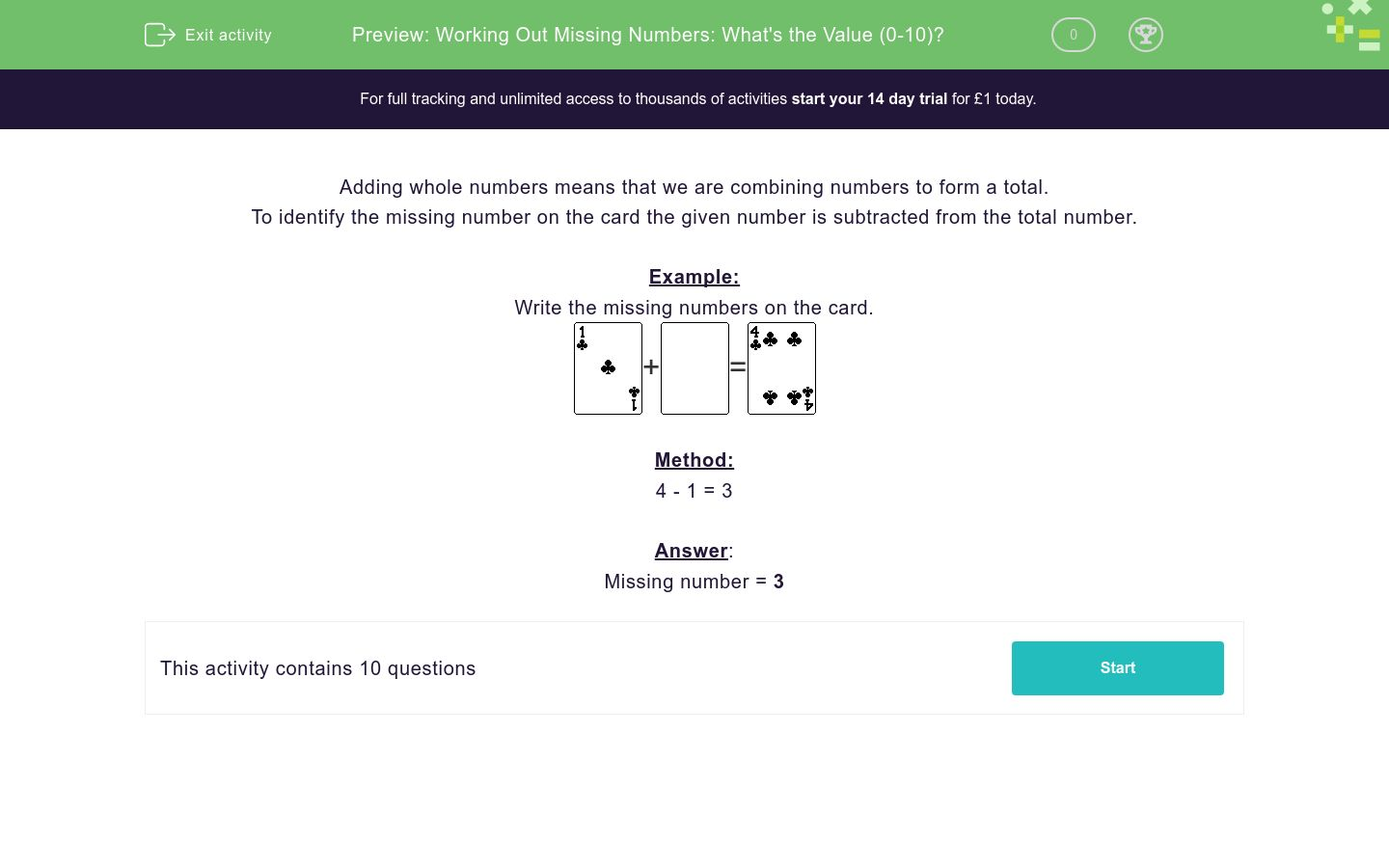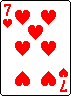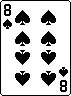# Working Out Missing Numbers: What's the Value (0-10)?

In this worksheet, students use their addition and subtraction skills to identify the missing numbers on the cards in the number range 0 to 10.Key stage:  KS 1

Curriculum topic:   Number: Addition and Subtraction

Curriculum subtopic:   Solve Add/Subtract Problems to 20

Difficulty level:### QUESTION 1 of 10

Adding whole numbers means that we are combining numbers to form a total.

To identify the missing number on the card the given number is subtracted from the total number.

Example:

Write the missing numbers on the card.+=Method:

4 - 1 = 3

Missing number = 3

Work out what number should be on the blank card and write the number in the answer box.+=Work out what number should be on the blank card and write the number in the answer box.+=Work out what number should be on the blank card and write the number in the answer box.+=Work out what number should be on the blank card and write the number in the answer box.+=Work out what number should be on the blank card and write the number in the answer box.+=Work out what number should be on the blank card and write the number in the answer box.+=Work out what number should be on the blank card and write the number in the answer box.+=Work out what number should be on the blank card and write the number in the answer box.+=Work out what number should be on the blank card and write the number in the answer box.+=Work out what number should be on the blank card and write the number in the answer box.+=• Question 1

Work out what number should be on the blank card and write the number in the answer box.+=3
EDDIE SAYS

Method

6 - 3 = 3

Missing Number = 3

• Question 2

Work out what number should be on the blank card and write the number in the answer box.+=2
EDDIE SAYS

Method

6 - 4 = 2

Missing Number = 2

• Question 3

Work out what number should be on the blank card and write the number in the answer box.+=4
EDDIE SAYS

Method

10 - 6 = 4

Missing Number = 4

• Question 4

Work out what number should be on the blank card and write the number in the answer box.+=5
EDDIE SAYS

Method

6 - 1 = 5

Missing Number = 5

• Question 5

Work out what number should be on the blank card and write the number in the answer box.+=3
EDDIE SAYS

Method

5 - 2 = 3

Missing Number = 3

• Question 6

Work out what number should be on the blank card and write the number in the answer box.+=4
EDDIE SAYS

Method

6 - 2 = 4

Missing Number = 4

• Question 7

Work out what number should be on the blank card and write the number in the answer box.+=3
EDDIE SAYS

Method

10 - 7 = 3

Missing Number = 3

• Question 8

Work out what number should be on the blank card and write the number in the answer box.+=7
EDDIE SAYS

Method

9 - 2 = 7

Missing Number = 7

• Question 9

Work out what number should be on the blank card and write the number in the answer box.+=1
EDDIE SAYS

Method

9 - 8 = 1

Missing Number = 1

• Question 10

Work out what number should be on the blank card and write the number in the answer box.+=6
EDDIE SAYS

Method

8 - 2 = 6

Missing Number = 6

---- OR ----

Sign up for a £1 trial so you can track and measure your child's progress on this activity.

### What is EdPlace?

We're your National Curriculum aligned online education content provider helping each child succeed in English, maths and science from year 1 to GCSE. With an EdPlace account you’ll be able to track and measure progress, helping each child achieve their best. We build confidence and attainment by personalising each child’s learning at a level that suits them.

Get started